In UML, relationships are connections between model elements. Use cases are also connected to each other in different kinds of relationships. The relationship between two use cases basically models the dependencies between two use cases. By reusing existing use cases using different types of relationships, the overall effort required to develop the system is reduced.

Use case diagrams show use cases, actors, and the relationships between them. For example, the relationship between an actor and a use case illustrates that the actor can use certain functionality of the business system.

Association Relationships

An association is a relationship between two classifiers, such as an actor and use cases, that describes the cause of the relationship and the rules that govern it. An association is a relationship between an actor and a business use case. It indicates that an actor can use the functionality of the business system.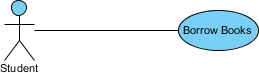#### Generalization Relationships

A generalization relation is a relationship in which one model element (child) is based on another model element (parent). Generalization relations are used in class diagrams, component diagrams, deployment diagrams, and use case diagrams to indicate that the child element accepts all the attributes, operations, and relationships defined in the parent element.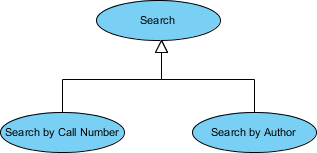#### Include Relationships

In UML modeling, a Include relationship is a relationship in which one use case (base use case) contains the functionality of another use case (inclusion use case). A containment relationship supports the reuse of functionality in the use case model.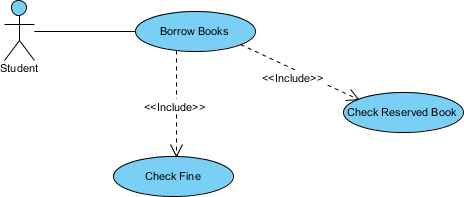#### Extending relationships

In UML modeling, you can use an extend relationship to specify that one use case (extension) extends the behavior of another use case (base). This type of relationship reveals details about the system or application that are usually hidden in the use case.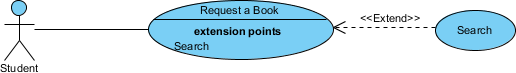More Use Case Examples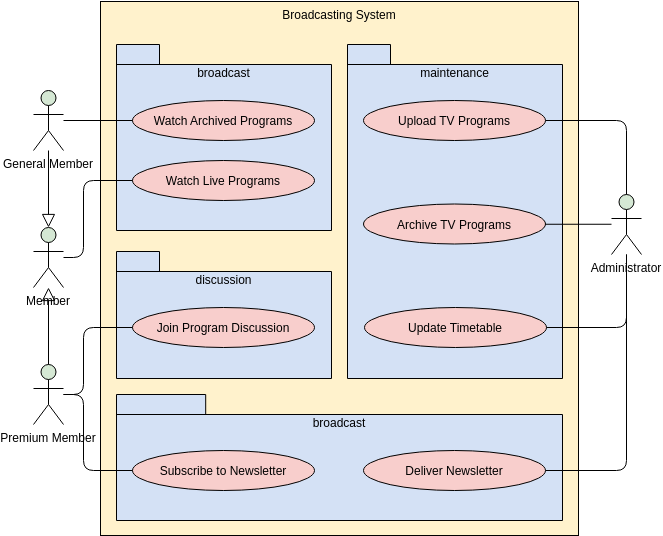Edit this Diagram

ATM Use Case Diagram Example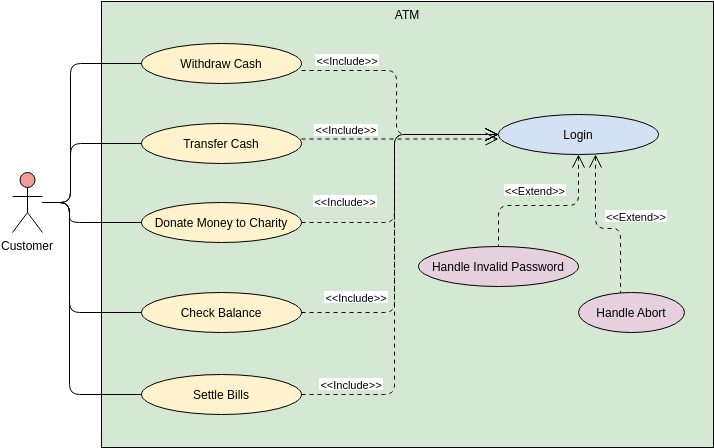Edit this Diagram

Use Case Diagram: Multiple Projects with System Boundaries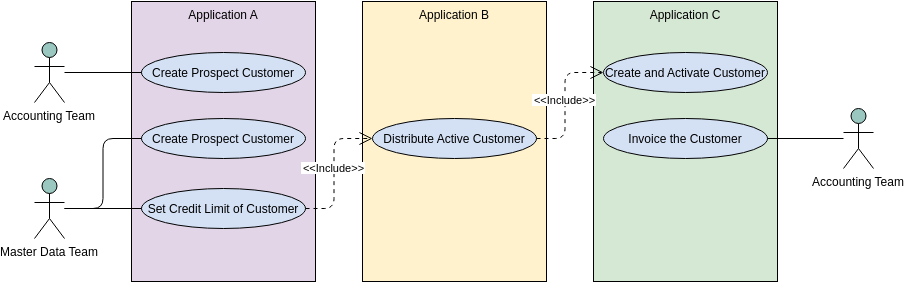Edit this Diagram

Use Case Diagram: Online Examination System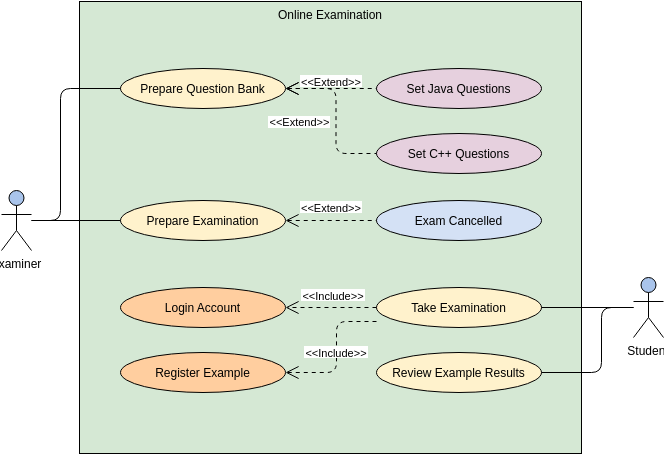Edit this Diagram

Use Case Diagram Example: Passenger ServiceEdit this Diagram

Use Case Diagram Example: Software Development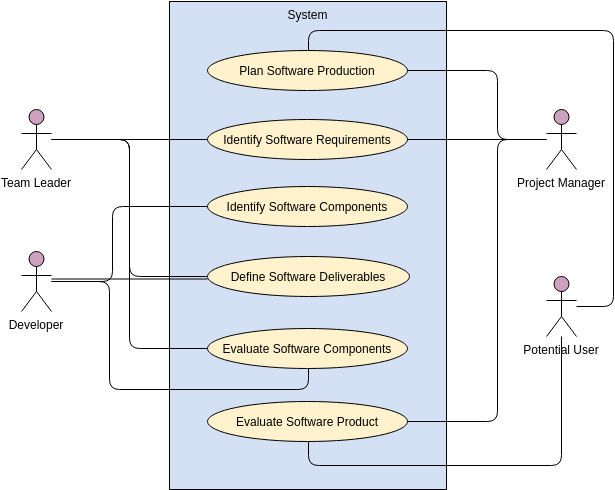Edit this Diagram

Use Case Diagram Example: Carpark System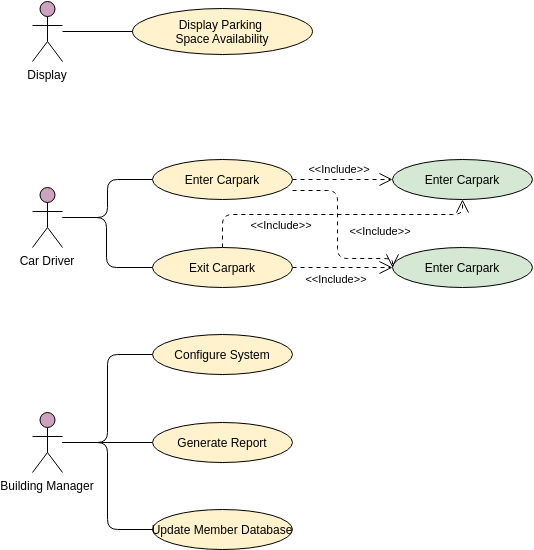Edit this Diagram

UML Use Case Diagram: Order Process System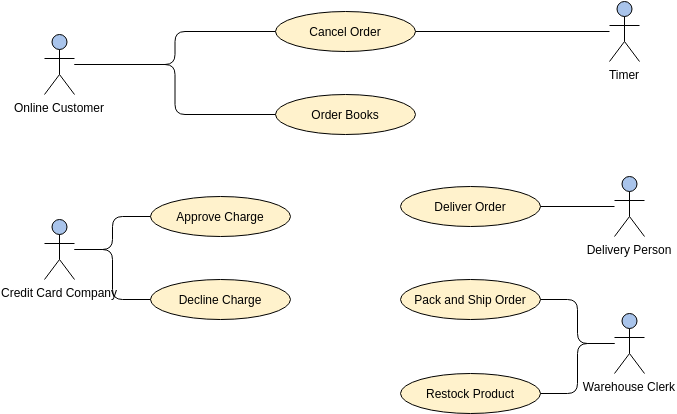Edit this Diagram

Include and Extend Use Case Diagram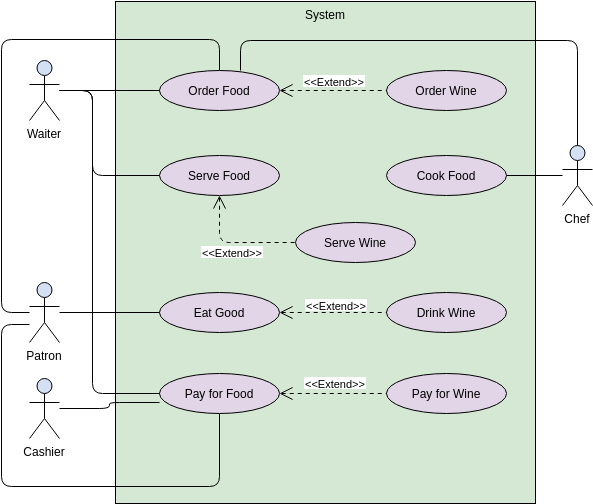Edit this Diagram

Use Case Diagram Example: Website (Extend and Include Use Case)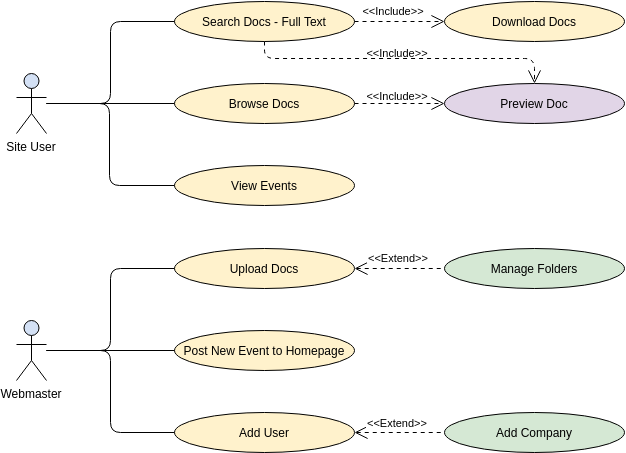Edit this Diagram

Use Case Diagram Example: External System as Actor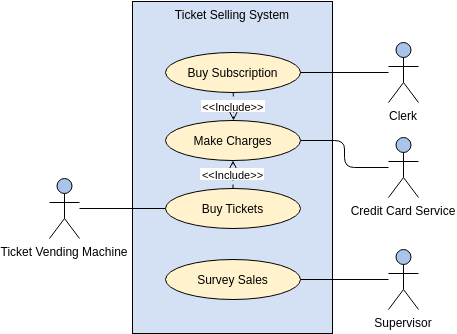Edit this Diagram

Use Case Diagram Example: Bank ATM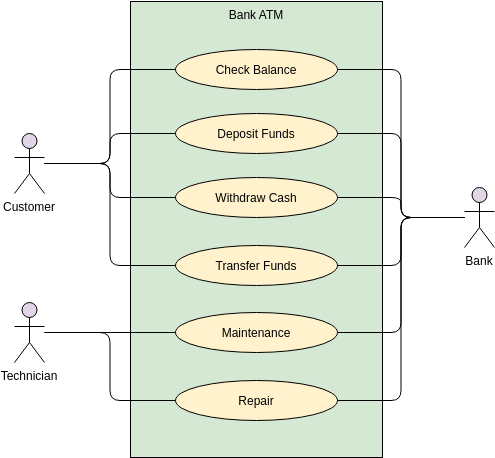Edit this Diagram

Use Case Diagram Example: Airport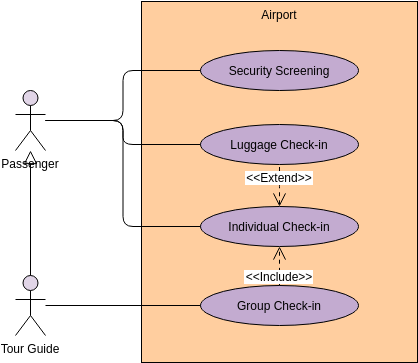Edit this Diagram